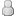• 11 Votes - 4.64 Average
• 1
• 2
• 3
• 4
• 5
12-10-2013, 05:07 PMPost: #127
 KillaMaakiJunior MemberPosts: 5 Joined: Nov 2013 Reputation: 0
```// Retro (CRT) shader, created to use in PPSSPP. #ifdef GL_ES precision mediump float; precision mediump int; #endif uniform sampler2D sampler0; varying vec2 v_texcoord0; uniform vec4 u_time; void main() {     // scanlines     int vPos = int( ( v_texcoord0.y + u_time.x * 0.5 ) * 272.0 );     float line_intensity = mod( float(vPos), 2.0 );          // color shift     float off = line_intensity * 0.0005;     vec2 shift = vec2( off, 0 );          // shift R and G channels to simulate NTSC color bleed     vec2 colorShift = vec2( 0.001, 0 );     float r = texture2D( sampler0, v_texcoord0 + colorShift + shift ).x;     float g = texture2D( sampler0, v_texcoord0 - colorShift + shift ).y;     float b = texture2D( sampler0, v_texcoord0 ).z;          vec4 c = vec4( r, g * 0.99, b, 1 ) * clamp( line_intensity, 0.85, 1 );          float rollbar = sin( ( v_texcoord0.y + u_time.x ) * 4 );          gl_FragColor.rgba = c + (rollbar * 0.02); }```